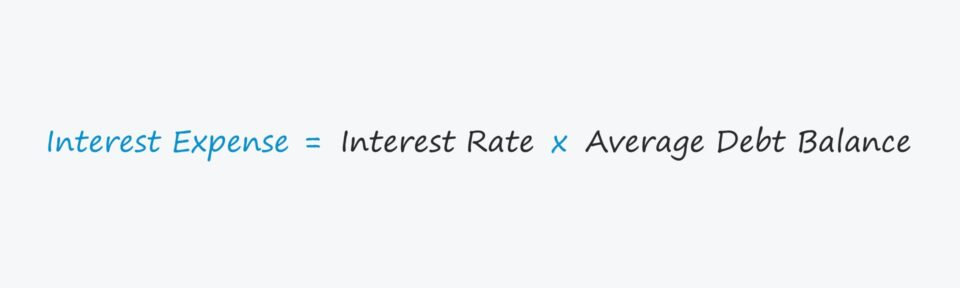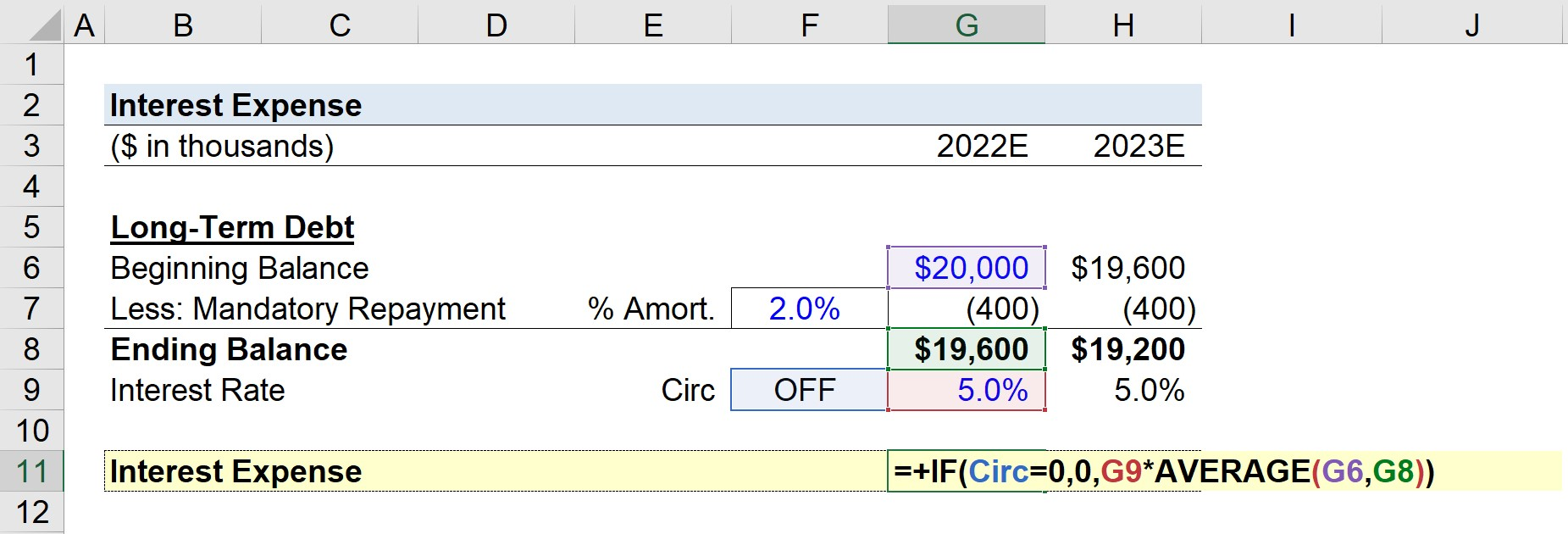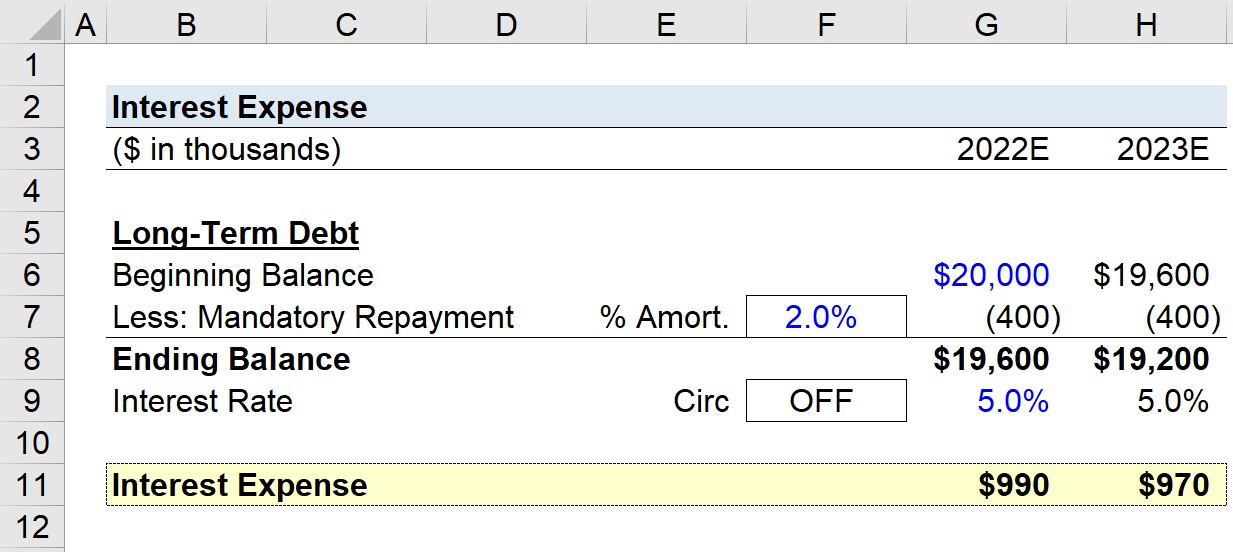Welcome to Wall Street Prep! Use code at checkout for 15% off.# Interest Expense

Guide to Understanding Interest Expense## Interest Expense: Accounting Definition

The interest expense line item appears in the non-operating section of the income statement, because it is a non-core component of a company’s business model.

The interest expense is often recorded as “Interest Expense, net”, meaning the company’s interest expense is net against its interest income, i.e. the income generated from short-term investments such as marketable securities.

The accounting treatment of interest expense is as follows:

• Income Statement (I/S) → On the income statement, interest expense impacts the earnings before taxes (EBT) line item, which effectively reduces the amount of taxes owed (i.e. the “interest tax shield”).
• Cash Flow Statement (CFS) → The cash flow statement does not directly capture interest expense, but interest expense is recorded on the income statement and affects the net income (“bottom line”). Therefore, interest is indirectly captured within the cash from operations (CFO) section, since net income is the starting line item of the cash flow statement (CFS)
• Balance Sheet (B/S) → The balance sheet is impacted by interest expense because of net income, which flows into the retained earnings line items on the balance sheet. Further, the interest incurred is determined by the outstanding principal on each debt instrument.

## How to Calculate Interest Expense?

To forecast interest expense in a financial model, the standard convention is to calculate the amount based on the average between the beginning and ending debt balances from the balance sheet.

In short, the amount of interest expense owed is a function of a company’s projected debt balances and the terms stated in the original lending arrangement.

The interest incurred is equal to the average debt principal balance multiplied by the applicable interest rate assumption, which can either be based on a fixed or floating rate (i.e. adjusts based on movements on an underlying benchmark rate).

## Modeling Interest Expense: Circularities from Average Debt

Interest expense is determined by a company’s average debt balance, i.e. the beginning and ending debt carrying amounts.

The problem, however, is that debt — namely, the revolving credit facility (i.e. “revolver”) — introduces a circularity into the financial model.

The circular reference, i.e. “circularity”, occurs when a formula references back to its own cell either directly or indirectly.

Forecasting interest using the average debt balance is the more intuitive approach, because a company repays debt over the borrowing term (and reduced principal directly results in less interest).

The greater the percentage of the original debt principal paid down over the borrowing term, the more the interest expense declines, all else being equal.

But to prevent a financial model from showing errors due to the endless loop of calculations – i.e. a “circularity” – a circularity switch is necessary, as we’ll soon demonstrate in our modeling tutorial.

Or, as an alternative solution, the beginning debt balance can also be used to avoid the circularity issue altogether.

## Interest Expense Formula

The formula for calculating the annual interest expense in a financial model is as follows.

Interest Expense = Interest Rate (%) x [(Beginning + Ending Debt Balance) / 2)]

For example, if a company has a total of \$100 million in debt at a fixed interest rate of 8%, the annual interest expense is calculated by multiplying the average debt principal by the interest rate.

Assuming there is no debt paydown during the year — i.e. the debt principal remains constant at \$100 million — the annual interest equals \$6 million.

• Annual Interest = \$100 × 6.0% = \$6 million

While the interest rate paid on specific borrowings can often be found in a public company’s filings, an alternative approach to estimate the interest rate is to divide the historical annual interest expense by the average debt balance on a weighted average basis.

## Interest Expense Calculator

We’ll now move to a modeling exercise, which you can access by filling out the form below.Submitting...

## 1. Long-Term Debt Financing Assumptions

Suppose a company decided to raise \$20 million in capital through issuances of loan with a long-term maturity near the end of 2021.

The ending balance in 2021 flows in as the beginning debt balance for the next year, 2022, in our long-term debt roll-forward schedule.

• Beginning 2022 Long-Term Debt Balance = \$20 million

Our simplified model assumes the mandatory repayment of the original principal is 2.0% per year.

Therefore, the principal amortization is calculated by multiplying the \$20 million debt balance by 2%, which is \$400k each year.

• % Required Amortization = 2% of Original Principal
• Mandatory Repayment = \$20 million * 2% = \$400k

The mandatory repayment reduces the ending debt balance, resulting in an ending balance of \$19.6 million at the end of 2022.

The \$19.6 million ending balance becomes the beginning balance for 2023, which is again reduced by the \$400k in principal repayment.

The ending long-term debt balance for 2023 is \$19.2 million — meaning that from the beginning of 2022 until the end of 2023, \$800k of the principal was paid off.

## 2. Interest Expense Calculation Example

Using the computed debt balances from the prior section, we’ll now calculate the interest expense owed by the borrower in each period.

Our interest rate assumption will be set at a fixed 5%, and we’ll create a circularity switch (and name it “Circ”).

• If “Circ” = 0 → Interest is Zero (Circularity Switch is “ON”)
• If “Circ” = 1 → Interest is Calculating Using the Average Debt Balance (Circularity Switch is “OFF”)

The formula in Excel for calculating interest is shown below.

=IF(Circ=0,0,Interest Rate*AVERAGE(Beginning Debt Balance,Ending Debt Balance))## 3. Interest Expense Schedule Build

Our hypothetical company’s annual interest expense is forecasted as \$990k in 2022, followed by an interest expense of \$970k in 2023.

• Forecasted Interest Expense, 2022E = \$990k
• Forecasted Interest Expense, 2023E = \$970k

In closing, the completed interest expense schedule from our modeling exercise illustrates the reduction in annual interest expense by \$20 million year-over-year (YoY) from 2022 to 2023, respectively.Step-by-Step Online Course

### Everything You Need To Master Financial Modeling

Enroll in The Premium Package: Learn Financial Statement Modeling, DCF, M&A, LBO and Comps. The same training program used at top investment banks.